Question

# Bill Blank signed an \$7,540 note at Citizen's Bank. Citizen's charges a 8.2% discount rate. Assume...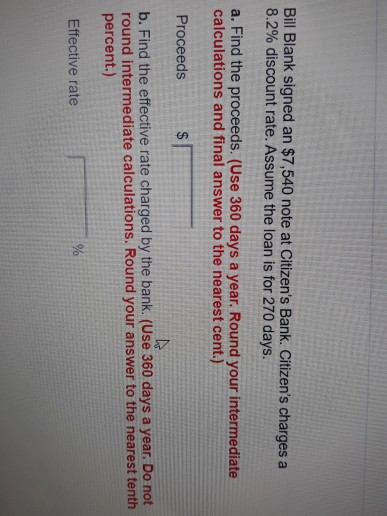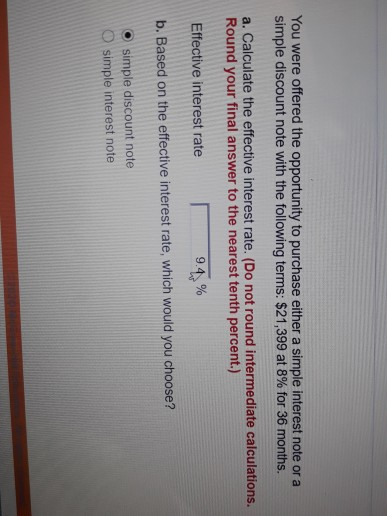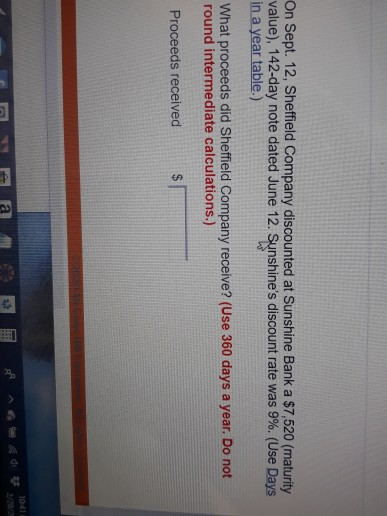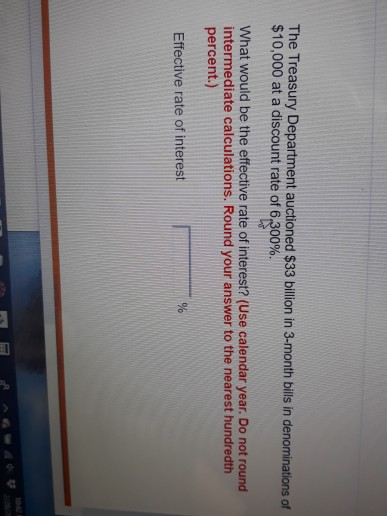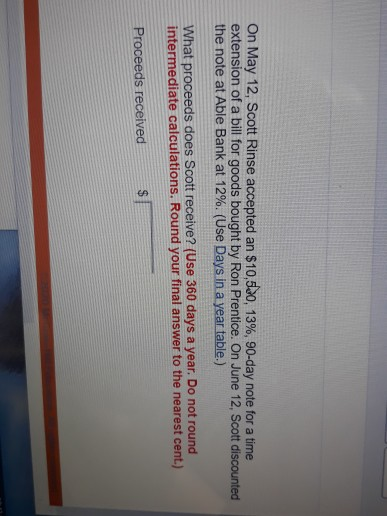Bill Blank signed an \$7,540 note at Citizen's Bank. Citizen's charges a 8.2% discount rate. Assume the loan is for 270 days. a. Find the proceeds. (Use 360 days a year. Round your intermediate calculations and final answer to the nearest cent.) Proceeds b. Find the effective rate charged by the bank. (Use 360 days a year. Do not round intermediate calculations. Round your answer to the nearest tenth percent.) Effective rate
You were offered the opportunity to purchase either a simple interest note or a simple discount note with the following terms: \$21,399 at 8% for 36 months. a. Calculate the effective interest rate. (Do not round intermediate calculations. Round your final answer to the nearest tenth percent.) Effective interest rate 9.4 % b. Based on the effective interest rate, which would you choose? • simple discount note simple interest note
On Sept. 12, Sheffield Company discounted at Sunshine Bank a \$7,520 (maturity value), 142-day note dated June 12. Sunshine's discount rate was 9%. (Use Days in a year table.) What proceeds did Sheffield Company receive? (Use 360 days a year. Do not round intermediate calculations.) Proceeds received \$ g ^ a 2 n
The Treasury Department auctioned \$33 billion in 3-month bills in denominations of \$10,000 at a discount rate of 6.300%. What would be the effective rate of interest? (Use calendar year. Do not round intermediate calculations. Round your answer to the nearest hundredth percent.) Effective rate of interest
On May 12, Scott Rinse accepted an \$10,5.00, 13%, 90-day note for a time extension of a bill for goods bought by Ron Prentice. On June 12, Scott discounted the note at Able Bank at 12%. (Use Days in a year table.) What proceeds does Scott receive? (Use 360 days a year. Do not round intermediate calculations. Round your final answer to the nearest cent.) Proceeds received

(a) Interest = \$7,540 * 0.082 * 270/360 = \$463.71

Proceed = \$7,540 - 463.71 = \$7,076.29

(b) Effective interest rate = 463.71 / (7,076.29 X 270/360) = 8.73%

#### Earn Coins

Coins can be redeemed for fabulous gifts.

Similar Homework Help Questions
• ### On May 20, Sheffield Company discounted at Sunshine Bank a \$7,290 (maturity value), 139-day note ...

On May 20, Sheffield Company discounted at Sunshine Bank a \$7,290 (maturity value), 139-day note dated Feb. 20. Sunshine’s discount rate was 8%. (Use Days in a year table.) What proceeds did Sheffield Company receive? (Use 360 days a year. Do not round intermediate calculations.) Proceeds received :?

• ### The face value of a simple discount note is \$4,000. The bank discount is calculated at...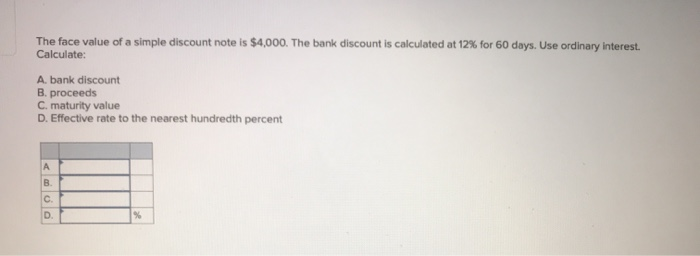The face value of a simple discount note is \$4,000. The bank discount is calculated at 12% for 60 days. Use ordinary interest. Calculate: A bank discount B. proceeds C. maturity value D. Effective rate to the nearest hundredth percent

• ### Solve for maturity value, discount period, bank discount, and proceeds.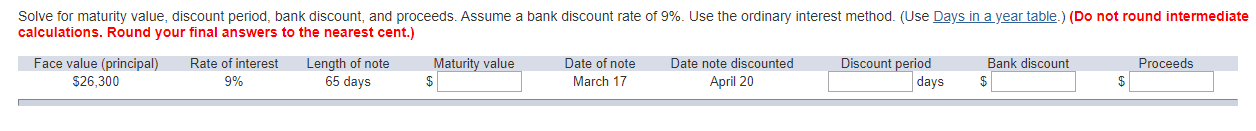Solve for maturity value, discount period, bank discount, and proceeds. Assume a bank discount rate of 9%. Use the ordinary interest method. (Use Days in a year table.) (Do not round intermediate calculations. Round your final answers to the nearest cent.)

• ### 8 On October 29, 2019, the Berkeley Company accepted a 60-day, 9 percent note from Devon...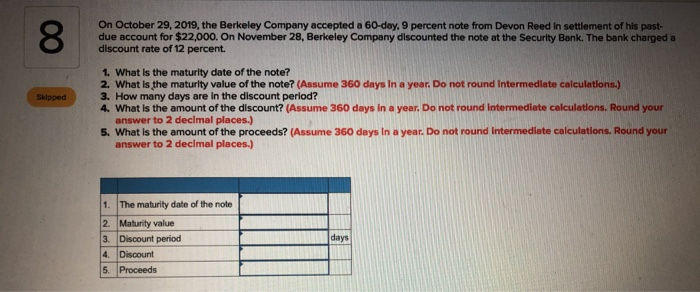8 On October 29, 2019, the Berkeley Company accepted a 60-day, 9 percent note from Devon Reed in settlement of his past- due account for \$22,000. On November 28, Berkeley Company discounted the note at the Security Bank. The bank charged a discount rate of 12 percent. 1. What is the maturity date of the note? 2. What is the maturity value of the note? (Assume 360 days in a year. Do not round Intermediate calculations.) 3. How many days...

• ### 11-8 Ron Prentice bought goods from Shelly Katz. On May 20, Shelly gave Ron a time...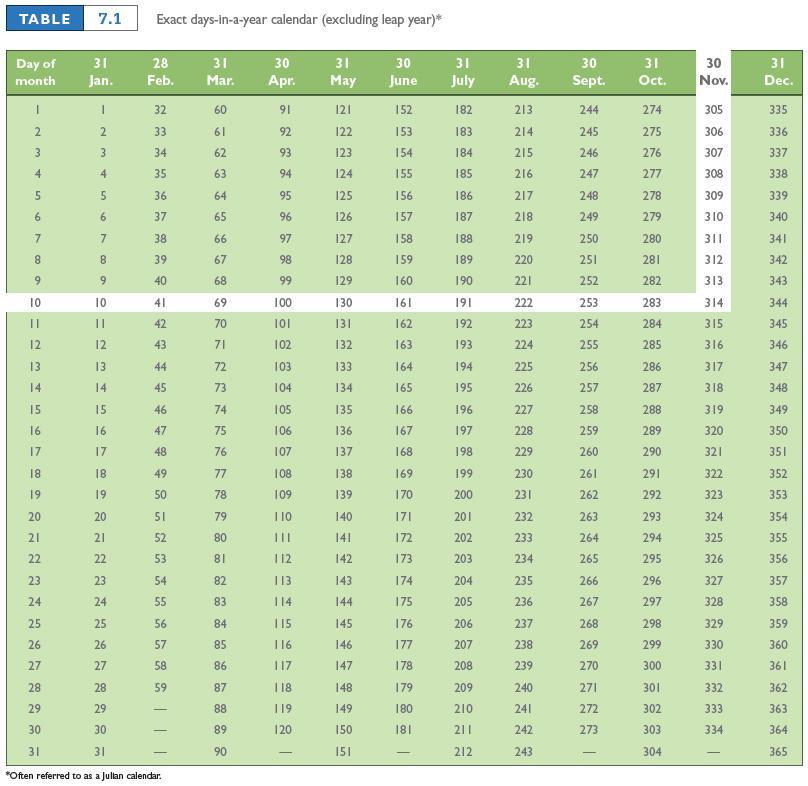11-8 Ron Prentice bought goods from Shelly Katz. On May 20, Shelly gave Ron a time extension on his bill by accepting a \$4,320, 6%, 175-day note. On Aug. 25, Shelly discounted the note at Roseville Bank at 8%. (Use Days in a year table.) What proceeds does Shelly Katz receive? (Use 360 days a year. Do not round intermediate calculations. Round your answer to the nearest cent.) Proceeds received            \$    11-9 Hafers, an electrical supply company, sold \$3,800 of...

• ### Sam Peters needs to calculate the effective interest rate of a simple discount note for \$3,600,...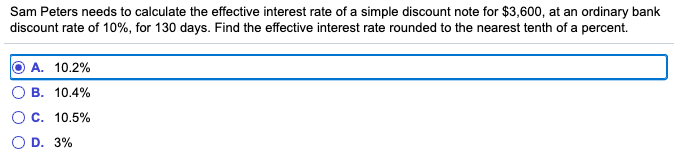Sam Peters needs to calculate the effective interest rate of a simple discount note for \$3,600, at an ordinary bank discount rate of 10%, for 130 days. Find the effective interest rate rounded to the nearest tenth of a percent. O A. 10.2% OB. 10.4% O C. 10.5% OD. 3%

• ### #9 Hafers, an electrical supply company, sold \$5,800 of equipment to Jim Coates Wiring, Inc. Coates...

#9 Hafers, an electrical supply company, sold \$5,800 of equipment to Jim Coates Wiring, Inc. Coates signed a promissory note May 12 with 3.0% interest. The due date was August 10. Short of funds, Hafers contacted Charter One Bank on July 20; the bank agreed to take over the note at a 4.7% discount. (Use Days in a year table.) What proceeds will Hafers receive? (Use 360 days a year. Do not round intermediate calculations. Round your final answer to...

• ### A man has a simple discount note for ​\$6,200​, at an ordinary bank discount rate of...

A man has a simple discount note for ​\$6,200​, at an ordinary bank discount rate of 8.53%, for 50 days. What is the effective interest​ rate using the banker's rule?

• ### Neveready Flashlights Inc. needs \$490,000 to take a cash discount of 2/10, net 60. A banker...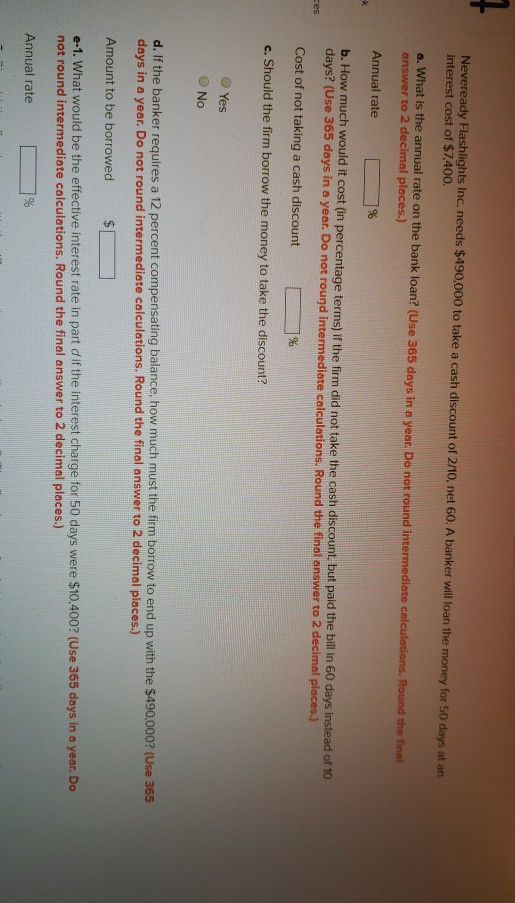Neveready Flashlights Inc. needs \$490,000 to take a cash discount of 2/10, net 60. A banker will loan the money for 50 days at an interest cost of \$7,400. a. What is the annual rate on the bank loan? (Use 365 days in a year. Do not round intermediate calculations. Round the final answer to 2 decimal places.) Annual rate % b. How much would it cost in percentage terms) ir the firm did not take the cash discount, but...

• ### Neveready Flashlights Inc. needs \$350,000 to take a cash discount of 3/18, net 72. A banker...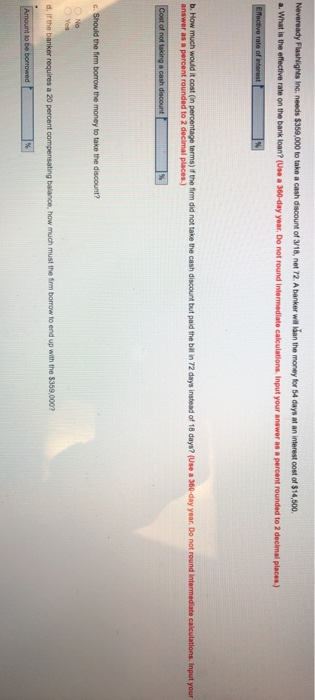Neveready Flashlights Inc. needs \$350,000 to take a cash discount of 3/18, net 72. A banker wil dan the money for 54 days at an interest cost of \$14,500 a. What is the effective rate on the bank loan? Use a 360-day year. Do not round Intermediate calculations. Input your answer as a percent rounded to 2 decimal places) Effective rate of interest b. How much would it cost in percentage terms) f the firm did not take the cash...